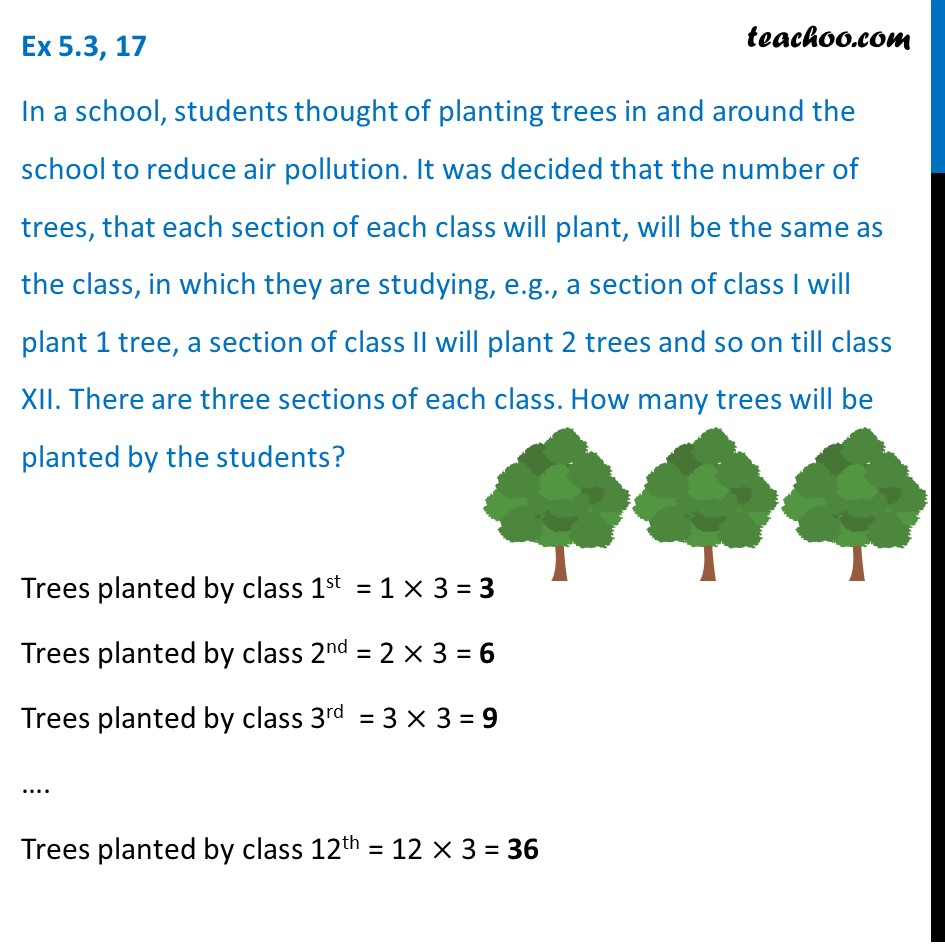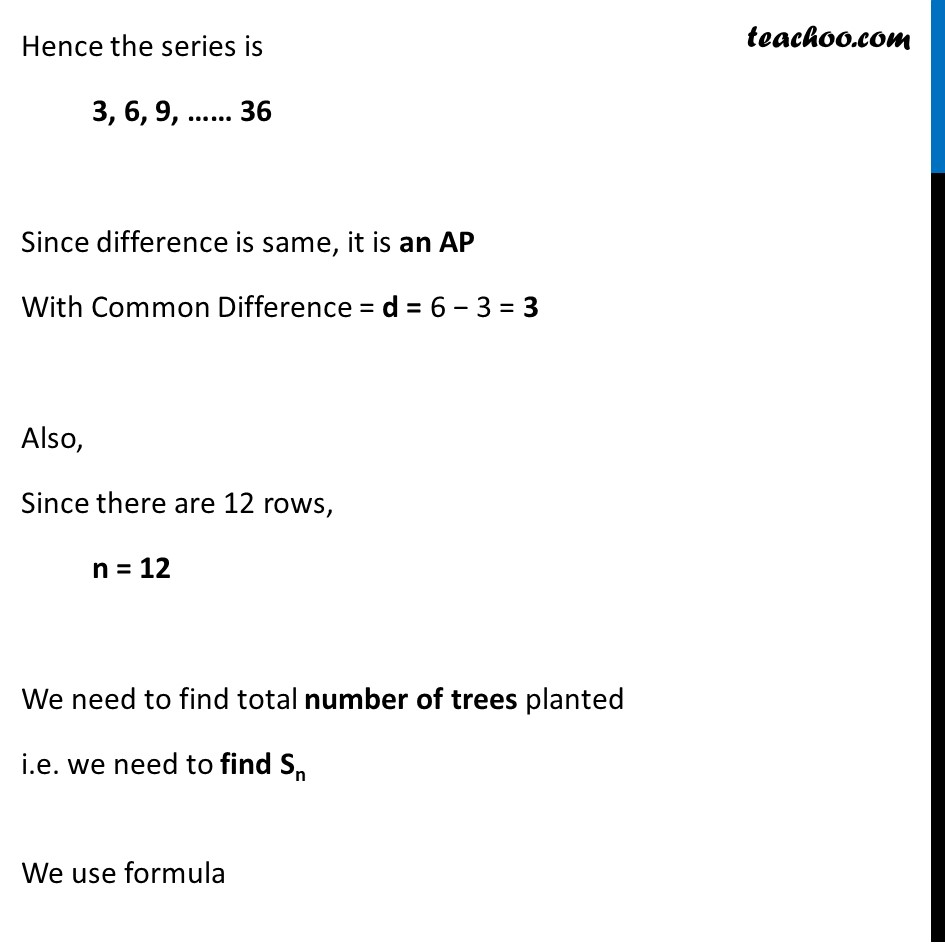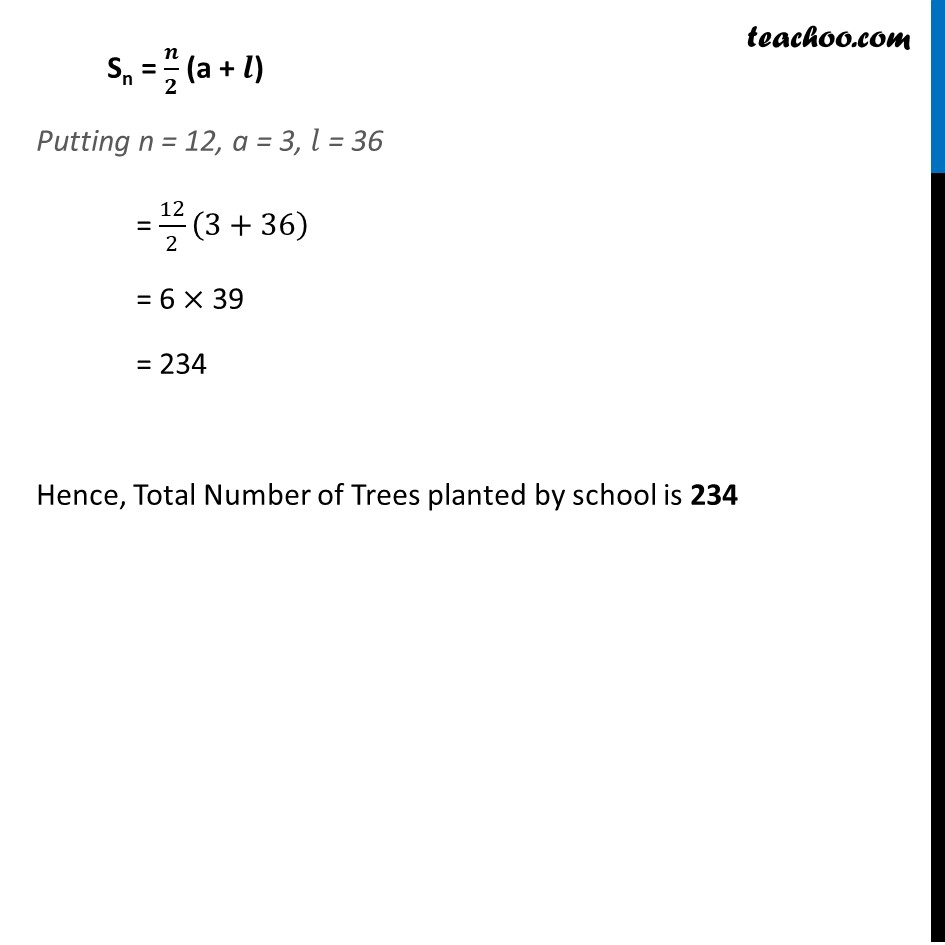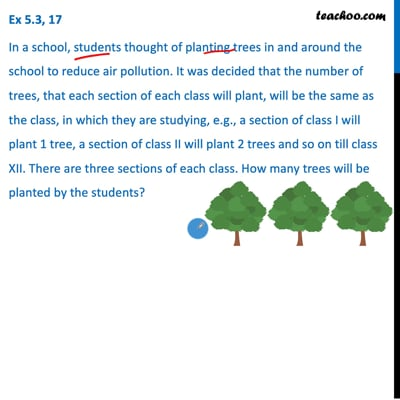Ex 5.3

Chapter 5 Class 10 Arithmetic Progressions
Serial order wiseThis video is only available for Teachoo black users

Solve all your doubts with Teachoo Black (new monthly pack available now!)

### Transcript

Ex 5.3, 17 In a school, students thought of planting trees in and around the school to reduce air pollution. It was decided that the number of trees, that each section of each class will plant, will be the same as the class, in which they are studying, e.g., a section of class I will plant 1 tree, a section of class II will plant 2 trees and so on till class XII. There are three sections of each class. How many trees will be planted by the students? Trees planted by class 1st = 1 × 3 = 3 Trees planted by class 2nd = 2 × 3 = 6 Trees planted by class 3rd = 3 × 3 = 9 …. Trees planted by class 12th = 12 × 3 = 36 Hence the series is 3, 6, 9, …… 36 Since difference is same, it is an AP With Common Difference = d = 6 − 3 = 3 Also, Since there are 12 rows, n = 12 We need to find total number of trees planted i.e. we need to find Sn We use formula Sn = 𝒏/𝟐 (a + 𝒍) Putting n = 12, a = 3, 𝑙 = 36 = 12/2 (3+36) = 6 × 39 = 234 Hence, Total Number of Trees planted by school is 234